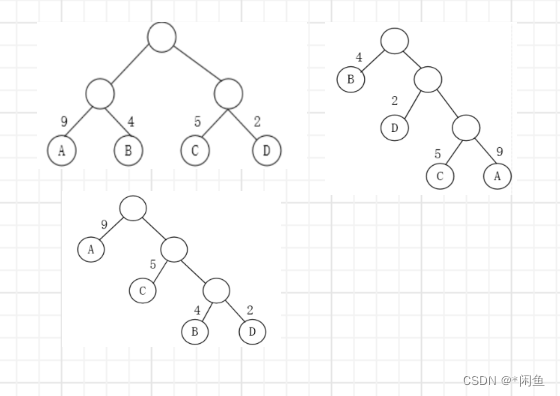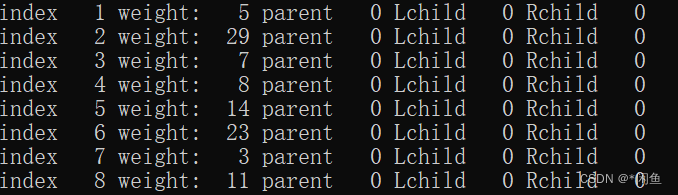0

# 赫夫曼树赫夫曼编码的创建

## 最优二叉树

WPL=(W1L1+W2L2+W3L3+…+WnLn)

WPL=24+34+43+92+72+82=80

2（9+4+5+2）=40
1
4+22+35+39=50
9
1+52+43+2*3=37## 如何构造赫夫曼树

• 根据给定的n个权值{w1,w2,……,wn}构成n棵二叉树的集合F={T1，T2……Tn}，其中每棵二叉树Ti中只有一个带权为wi的根结点，其左右子树均为空。
• 在F中选取两棵根节点的权值最小的树作为左右子树构造一棵新的二叉树，且置新的二叉树的根节点的权值为其左，右子树上根节点的权值之和
• 在F中删除这棵树，同时将新得到的二叉树加入F中。
• 重复2，3步骤，直到F只含一棵树为止，这棵树便是赫夫曼树。## 编码与压缩文件

• 统计文件中所有字符出现的频次
• 将字符作为节点的值，字符出现的频次作为节点的权，构建哈夫曼树
• 将哈夫曼树的每个节点的字符与对应的 01 编码作为映射存储到字典中
• 再次读取文件，将每个字符转化为 01 编码的字符串格式
• 将这个文件对应的 01 编码字符串转换为真正的比特并写入到文件中，完成压缩

• 读取压缩文件，将压缩文件转换为 01 的字符串形式
• 将哈夫曼树的每个节点对应的 01 编码与字符值作为映射存储到字典中（HashMap）
• 对压缩文件转换成的字符串进行遍历；使用两个指针 i，j，第一个指针 i 指向起始点，第二个指针 j 以 i 所在的位置开始从左向右逐一扫描。如果 i～j 对应的字符串在字典中有匹配的 key，那么就在字典中取出对应的 value 写入到还原的文件中，并更新指针 i 的位置；无匹配的 key 则继续移动 j 指针直至匹配。当 i 移动到字符串最后的位置时，结束遍历，解压完成。

## 结构体设计

``````#pragma onceconstint n =8;constint m = n *2;typedefunsignedint WeigthType;typedefunsignedint NodeType;typedefstruct{
WeigthType weight;
NodeType parent, leftchild, rightchild;}HTNode;typedef HTNode HuffManTree[m];typedefstruct{char ch;char code[n +1];}HuffCodeNode;typedef HuffCodeNode HuffCoding[n +1];struct IndexWeigth
{int index;
WeigthType weight;operatorWeigthType()const{return weight;}};
``````

## 创建赫夫曼树

``````voidInitHuffManTree(HuffManTree hft, WeigthType *w){memset(hft,0,sizeof(HuffManTree));for(int i =0; i < n; i++){
hft[i+1].weight = w[i];}}voidPrintHuffManTree(HuffManTree hft){for(int i =1; i <m;++i){printf("index %3d weight: %3d parent %3d Lchild %3d Rchild %3d\n",i,hft[i].weight,hft[i].parent,hft[i].leftchild,hft[i].rightchild);}}voidCreateHuffManTree(HuffManTree hft){
priority_queue<IndexWeigth, vector<IndexWeigth>, std::greater<IndexWeigth>>qu;for(int i =1; i <= n;++i){
qu.push(IndexWeigth{i,hft[i].weight});}int k = n +1;while(!qu.empty()){if(qu.empty())break;
IndexWeigth left = qu.top(); qu.pop();if(qu.empty())break;
IndexWeigth right = qu.top(); qu.pop();
hft[k].weight = left.weight + right.weight;
hft[k].leftchild = left.index;
hft[k].rightchild = right.index;
hft[left.index].parent = k;
hft[right.index].parent = k;
qu.push(IndexWeigth{k,hft[k].weight});
k +=1;}}
``````

## 创建构建赫夫曼编码

``````voidInitHuffManCode(HuffCoding hc,char* ch){memset(hc,0,sizeof(HuffCoding));for(int i =1; i <= n;++i){
hc[i].ch = ch[i-1];
hc[i].code='\0';}}voidPrintHuffManCode(HuffCoding hc){for(int i =1; i <= n;++i){printf("data: %c => code : %s \n", hc[i].ch, hc[i].code);}}voidCreateHuffManCode(HuffManTree hft, HuffCoding hc){char code[n +1]={0};for(int i =1; i <= n;++i){int k = n;
code[k]='\0';int c = i;int pa = hft[c].parent;while(pa !=0){
code[--k]= hft[pa].leftchild==c?'0':'1';
c = pa;
pa = hft[c].parent;}strcpy_s(hc[i].code,n,&code[k]);}}
``````

### “赫夫曼树赫夫曼编码的创建”的评论:

##### 关于作者##### overfit同步小助手